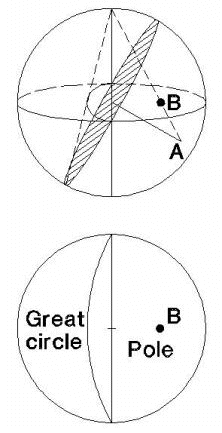The Rocscience International Conference 2021 Proceedings are now available. Read Now

# Equal Angle Projection

For the Equal Angle projection method, a line is drawn from the center of the top of the sphere (the zenith), to a point A on the sphere (a pole or a point on the great circle). The intersection of this line with a horizontal plane through the center of the sphere, defines the projection point B.

Equal Angle Projection methodSee the Projection Examples topic for a comparison of plots using Equal Angle and Equal Area projection.